# Definition of circle Circumference, Area, Chord, Segment

## Definition of circle

When a line AB rotate by 360 degree taking center A, thus obtained figure is called circle.

A is called center of circle. A to any point on the circle joining is called radius, a line passing through the center of the circle is called diameter of circle and its value is 2 * radius.

The ratio between circumference to diameter is always constant and denoted by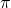its value isor 3.14 approximately.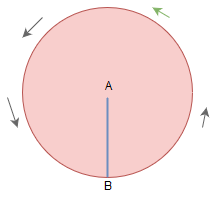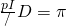## Circumference of circle

so circumference of circle (p)=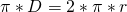## Area of circle formula

Area of circle==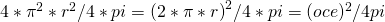We divide a circle in to many small sectors, thus the length of an arc is reached near about the length of chord.

Then merge them as below we have obtained a ractangle.

## Length of ChordLength of chord=where 2a=lengh of chord
h= height of arclength of arc= arc/oce=Angle in degree/360
Radion: If are is equal in length to the radious, then the angle for form are called radion.

## Segment of a circle

chord AB of a circle divides the circular region into two part, each part is called a segment of the circle.Sector of the circle– the area surrounded by an arc and the two radius joining the end point of the arc with the center is called a sector of the circle.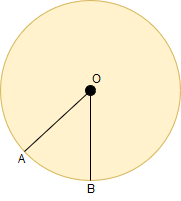Concentric circle– two or more circles with the given centre are called concentric circle.